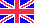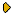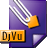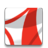Журнал Экспериментальной и Теоретической Физики
 НАЧАЛО | ПОИСК | ДЛЯ АВТОРОВ | ПОМОЩЬОбщая информация о журналеЗолотые страницыАдреса редакцииСодержание журналаСообщения редакцииПравила для авторовЗагрузить статьюПроверить статус статьиЖЭТФ, Том 146, Вып. 1, стр. 87 (Июль 2014)
(Английский перевод - JETP, Vol. 119, No 1, p. 75, July 2014 доступен on-line на www.springer.com )

PILGRIM DARK ENERGY IN f(T) GRAVITY

Поступила в редакцию: 3 Февраля 2014

DOI: 10.7868/S0044451014070104DJVU (114K)PDF (284.3K)

We discuss the interacting f(T) gravity with pressureless matter in an FRW spacetime. We construct an f(T) model by following the correspondence scheme incorporating a recently developed pilgrim dark energy model and taking the Hubble horizon as the IR cutoff. We use constructed model to discuss the evolution trajectories of the equation-of-state parameter, the ωT-ω'T phase plane, and state-finder parameters in the evolving universe. It is found that the equation-of-state parameter gives a phantom era of the accelerated universe for some particular range of the pilgrim parameter. The ωT-ω'T plane represents freezing regions only for an interacting framework, while the ΛCDM limit is attained in the state-finder plane. We also investigate the first and second laws of thermodynamics assuming equal temperatures at and inside the horizon in this scenario. Due to the violation of the first law of thermodynamics in f(T) gravity, we explore the behavior of the entropy production term. The validity of a generalized second law of thermodynamics depends on the present-day value of the Hubble parameter.

Сообщить о технических проблемах